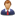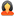Question

All Subjects Biology General Biology Bio156 lab 1

Offered Price \$ 30.00

Bio156 lab 1

Question # 00003558
Subject: Biology
Topic: General Biology
Due on: 11/28/2013
Posted On: 11/14/2013 06:09 PM

Rating:
4.1/5Posted ByQuestions:
4867
Tutorials:
6693
Feedback Score:

Question

LAB 1

1.Describe the function of the following pieces of safety equipment and how each might be used: (10 points)

a.Eye Wash (2 points)

b.Fire Blanket (2 points)

c.Shower (2 points)

d.Chemical Spill Kit (2 points)

e.Biological Spill Solution (2 points)

2.Briefly describe a situation when each of the following waste disposal containers would be used and give examples of the waste involved: (4 points)

a.Broken Glass Container (2 points)

b.Biological Hazards Materials Container (2 points)

3.Metric Measurements: Perform each of the following conversions. (24 points)

a.Given that one inch = 2.54 cm. State the student's height in inches and then convert to centimeters. (4 points)

b.The width of a normal sheet of paper is 8.5 inches. Convert this length to kilometers. (4 points)

c.A lab exercise in BIO156 required 300 ml of water that was poured from a two-liter container. How many milliliters were left in the original container? (4 points)

d.A typical can of soda is 355 ml. How many liters are there in this can of soda? (4 points)

e.The weight of a small bag of sugar is 2.265 kg. The student is baking cakes for a college scholarship fundraiser. It takes 100 grams of sugar to make one small cake. How many cakes can be made from a single bag of sugar? (4 points)

f.The student is conducting a nutrition experiment using lab rats. One group (control group) is fed a normal diet, while the other group (experimental group) is fed a diet high in protein. There were 30 rats total used in the experiment. At the end of the experiment, the average mass (weight) of a rat in the control group was 60.4 g while the average mass (weight) of a rat in the experimental group was 0.0689 kg. What is the average difference in weight between the two groups in grams? (4 points)

4.Temperature: Perform the following temperature conversions: (6 points)

a.Normal body temperature is 98.6°F. The student is not feeling well and only has a Celsius thermometer available. The student's temperature is taken and the thermometer reads 38.5°C.

1.Convert the temperature to Fahrenheit. (1 point)

2.Is the student running a fever (i.e., is the normal body temperature above normal)? (1 point)

b.The student's cookbook says roast beef is rare at 140°F, medium at 160°F, and well done at 170°F. The student prefers beef cooked medium and only has a meat thermometer in °C. What temperature will the thermometer reach for the roast to be done the way the student likes it? (2 points)

c.The student is driving in Mexico and notices that daytime temperatures are only given in °C. The student sees a sign indicating that the current temperature is 29°C. What is the temperature in °F? (2 points)

5.What is the purpose of the control group in the Scientific Method? Briefly explain and use examples. (5 points)

6.Design a (hypothetical) experiment that adheres to the Scientific Method. Be sure to include all the necessary requirements at each step and give examples at all of the steps. Start with an observation, whether it's real or made up, state the null hypothesis, and design an experiment (including an experimental and control group, random sampling, sample size, and reproducibility) that will allow the student to reject or fail to reject the hypothesis, and state the conclusion (** 20 points.)

**Note that the point value of this question is 20 points. It is imperative that one thoroughly understands and can apply the Scientific Method as it is necessary to the understanding of all science. Take the time to ensure the answer is thorough, inclusive, and complete.

7.

a.What solution is used to clean biological spills (be specific)? (2 points)

b.Why is this different than solutions used for chemical spills? (3 points)

8.List three things that one can do to protect oneself from contamination/injury in the laboratory. (6 points)

a.(2 points)

b.(2 points)

c.(2 points)

9.(Application) How might the information gained from this lab pertaining to the scientific method, safety, or measurement be useful to a student employed in a healthcare related profession? (20 points)

Tutorials for this Question
Available for
\$ 10.00

Bio156 lab 1

Tutorial # 00003363
Posted On: 11/14/2013 07:30 PM
Posted By:neil2103Questions:
9877
Tutorials:
14914
Feedback Score:
Tutorial Preview … …
Attachments
Bio156_LAB_1.docx (16.93 KB)
Preview: of a rat in the experimental group was 0.0689 kg. What is the average difference in weight between the two groups in grams? (4 points)64.65 kg4.Temperature: Perform the following.....
* - Additional Paypal / Transaction Handling Fee (3.9% of Tutorial price + \$0.30) applicable